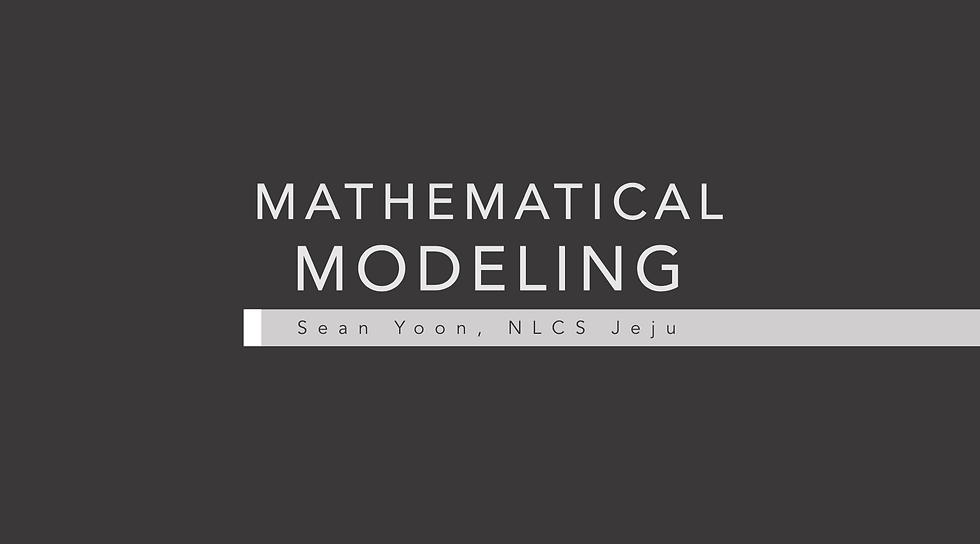top of page
All Posts: Blog2

# [Math Circle] Mathematical Modelling Presentation in OpenSchool

Updated: Nov 2, 2020

At the moment, I am involved in a project named "Open school" in the KGSEA math circle. The aim of the project is to record and upload online lectures of intermediate mathematics so that students without access to advanced math education could also easily learn such content. While we mostly focus on olympiad mathematics, we also have "special lectures" where we record videos around 30 minutes about topics that each of us is interested in. We present the lectures during our regular meetings, so each of us can also learn from them. This month, I had been nominated as the lecturer.

And if you've read any posts from the "STEM and Research" section of my blog, yes--I presented about my favourite part in STEM, which is mathematical modelling. The concept of mathematical modelling is extremely broad--after a long pondering of what content to include within my thirty minutes, I decided to make the content as intuitively possible to understand as possible.

I recalled when I first met the concept of "mathematical modelling"--I was even unclear what the word meant. Hence, I put in several slides to contextualize the word and provide some examples. Then, I gave the formal definition and explained why the examples fit into that definition.

The next part was about, then, how to make such models. I introduced the 7 steps and mapping from the real world to the mathematical world. Also, I talked about the whitebox and blackbox method, multi-model prediction, the Condorcet Jury theorem, and the Diversity prediction theorem. I finally gave an example of constructing a model through these 7 steps, by giving a hypothetical predator-prey interaction in an isolated island. More specifically, I generalized this situation into a Lotka-Volterra model, and talked about how such models could be extended to virology.I put a lot of effort into the design of the ppt. It took far longer than I expected due to the design. You can download it on the link below.

KGSEA Presentaiton
.pdf